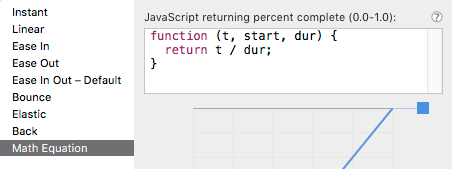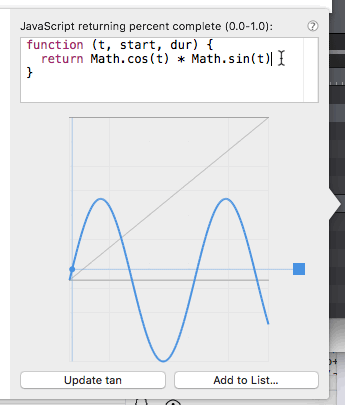# Math Equation in Easing Functions, how-to?

HI to all JavaScript-savvy beta testers!

Does anyone have an example or a visual how-to post about that new Math Equation?Visually Yours,
Ed

At this point in the Keynote, Jonathan does a quick demo (a bit hard to see): https://youtu.be/d_PKAJJhRoM?t=23m44s

So in the sample, the default is:

`return t / dur`

So you’re creating a function that charts the course of an element using these variables:

• `t` The absolute time in the timeline (floating point number)
• `start` is the time of the initial keyframe (floating point number)
• `dur` the total duration of the animation as a floating point number.

To get the current time of the animation regardless of its start point in your timelines, you can use (t - start)

I realized that you can create a randomness emitter over a animation path to plot random positions using `Math.random(t)` :

3 Likes

This page that @Jonathan shared has a great set of functions: https://soulwire.co.uk/math-for-motion/

For example:You can use this in Hype by writing out

`return Math.cos(t) * Math.sin(t)`

If the curves are too steep, you can multiply it by a number smaller than 1:cos sin equation.zip (19.3 KB)

5 Likes

Many thanks to @Daniel for those great visual Math Equations explainers.